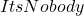## If the kinetic energy of the ball decreases then the potential energy will￼____

Question

If the kinetic energy of the ball decreases then the potential energy will￼____

in progress 0
6 months 2021-07-30T15:42:01+00:00 1 Answers 19 views 0

## Answers ( )

1. Hey There!! ~

The answer to this is: Complete these sentences.

If a bouncing ball has a total energy of 20 J and a kinetic energy of 5 J, the ball’s potential energy is 15J.

If the kinetic energy of the ball decreases, then the potential energy will Increase.

Hope It Helped!~~